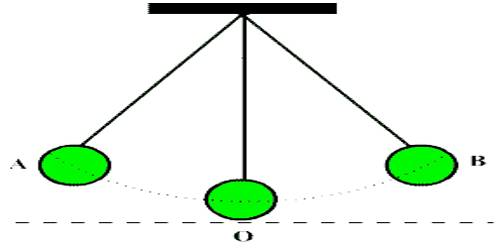# Characteristics of Periodic Motion

Characteristics of periodic motion:

Characteristics of periodic motion are the velocity of the object, the period of motion and the amplitude of the motion. Periodic motion is seen in different waveforms and is used in clocks and other devices. Time taken by a particle, having periodic motion, for passing particular point from a particular direction at regular interval is called time period. Path of a periodic motion can be circular, elliptical, linear or more complex.

Periodic motion has three similar characteristics: velocity, period, and amplitude.

Velocity: They all have a velocity. You can evaluate the velocity of a bouncing ball, the weight on a pendulum, or such.

Period: The period is the time the object takes to go back and forth. If you spin a weight on a string, you can determine the time it takes to go 1 revolution. Drop a ball and measure the time it takes until it bounces back up. That is its period.

Amplitude: The amplitude is 1/2 the distance the object goes before from one side of the period to the other. For an object in the rotation, the amplitude is the radius of the circle (1/2 the diameter). The amplitude of a pendulum swing would be the distance from the bottom to the height on one side of the swing.

Periodic motion is when the motion of an object continually repeats itself, such as repeatedly moving back and forth or moving in a circular orbit. Again let consider a thread is kept randomly on the floor. An ant is moving over that thread with a uniform speed. It is seen that the ant is passing through a fixed point of the thread at a regular interval. Here the thread has no fixed geometrical shape. Even then the motion of the ant will be periodic motion. The motion of strings of musical instruments or motion of the atmosphere, the motion of the needle of a clock, the motion of the piston of a cylinder of the steam or petrol engines, oscillation of atoms in the solid body etc. are periodic motion.